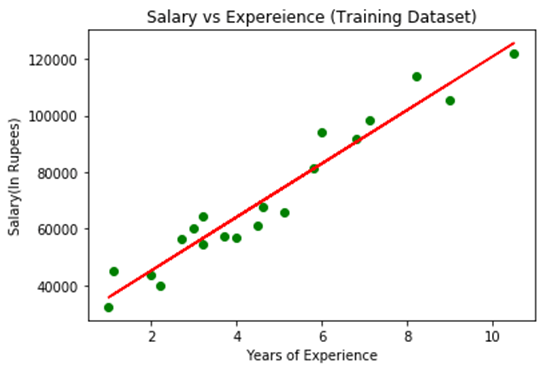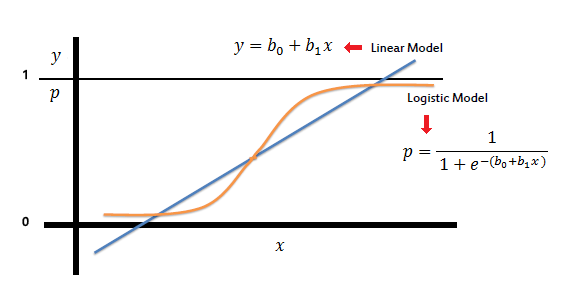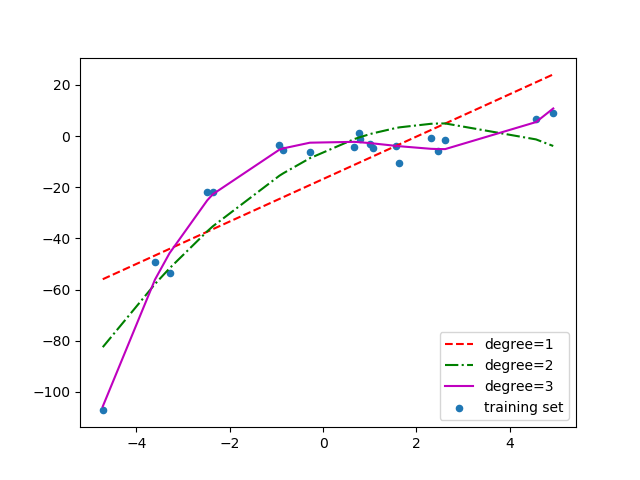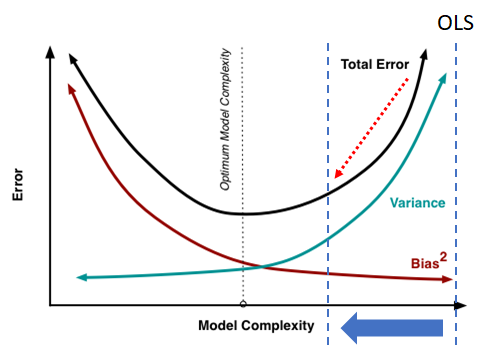# Regression techniques in Machine Learning

By Learnbay Category Machine Learning Reading time 7-8 mins Published on May 29, 2022

# Regression techniques in Machine Learning

Machine learning has become the sexiest and very trendy technology in this world of technologies, Machine learning is used every day in our life such as Virtual assistance, for making future predictions, Videos surveillance, Social media services, spam mail detection, online customer support, search engine resulting prediction, fraud detection, recommendation systems, etc. In machine learning, Regression is the most important topic that needed to be learned. There are different types of Regression techniques in Machine Learning which we will know in this article.

## Introduction

Regression techniques in Machine Learning such as Linear regression and Logistic regression are the most important algorithms that people learn while they study about Machine learning algorithms. There are numerous forms of regression that are used to perform regression and each has its own specific features, that are applied accordingly. The regression techniques are used to find out the relationship between the dependent and independent variables or features. It is a part of data analysis that is used to analyze the infinite variables and the main aim of this is forecasting, time series analysis, modeling.

## What is Regression?

Regression is a statistical method that mainly used for finance, investing and sales forecasting, and other business disciplines that make attempts to find out the strength and relationship among the variables.

There are two types of the variable into the dataset for apply regression techniques:

Dependent Variable that is mainly denoted as Y Independent variable that is denoted as x.

And, There are two types of regression

Simple Regression: Only with a single independent feature /variable Multiple Regression: With two or more than two independent features/variables.

Indeed, in all regression studies, mainly seven types of regression techniques are used firmly for complex problems.

• Linear regression
• Logistics regression
• Polynomial regression
• Stepwise Regression
• Ridge Regression
• Lasso Regression

## Linear regression

It is basically used for predictive analysis, and this is a supervised machine learning algorithm. Linear regression is a linear approach to modeling the relationship
between scalar response and the parameter or multiple predictor variables. It focuses on the conditional probability distribution. The formula for linear regression is Y = mX+c.

Where Y is the target variable, m is the slope of the line, X is the independent feature, and c is the intercept.

## Additional points on Linear regression

1. There should be a linear relationship between the variables.
2. It is very sensitive to Outliers and can give a high variance and bias model.
3. The problem of occurring multi colinearity with multiple independent features

### Logistic regression

It is used for classification problems with a linear dataset. In layman’s term, if the depending or target variable is in the binary form (1 0r 0), true or false, yes or no. It is better to decide whether an occurrence is possibly either success or failure.1. It is used for classification problems.
2. It does not require any relation between the dependent and independent features.
3. It can after by the outliers and can occur underfitting and overfishing.
4. It needs a large sample size to make the estimation more accurate.
5. It needs to avoid collinearity and multicollinearity.

### Polynomial regression

The polynomial regression technique is used to execute a model that is suitable for handling non-linear separated data. It gives a curve that is best suited to data points, rather than a straight line.

The polynomial regression suits the least-squares form. The purpose of an analysis of regression to model the expected y value for the independent x of the dependent variable.

The formula for this Y= β0+ β0x1+eLook particularly for curve towards the ends to see if those shapes to patterns make logical sense. More polynomials can lead to weird extrapolation results.

### Step-wise Regression

It is used for statistical model fitting regression with predictive models. It is done automatically.

The variable is supplemented or removed from the explanatory variable set at every step. The main approaches for the regression are reverse elimination and bidirectional elimination and step by step approaches.

The formula for this: b = b(sxi/sy)

1. This regression provides two things, the very first one is to add prediction for each steep and remove predictors fro each step.
2. It starts with the most significant predictor into the ML model and then adds features for each step.
3. The backward elimination starts with all the predictors into the model and then removes the least significant variable.

### Ridge Regression

It is a method that used when the dataset having multicollinearity which means, the independent variables are strongly related to each other. Although the least-squares estimates are unbiased in multicollinearity, So after adding the degree of bias to the regression, ridge regression can reduce the standard errors.1. In this regression, normality is not to be estimated the same as Least squares regression.
2. In this regression, the value could be varied but doesn’t come to zero.
3. This uses the l2 regularization method as it is also a regularization method.

### Lasso Regression

Lasso is an abbreviation of the Least Absolute shrinkage and selection operator. This is similar to the ridge regression as it also analyzes the absolute size of the regression coefficients. And the additional features of that are it is capable of reducing the accuracy and variability of the coefficients of the Linear regression models.